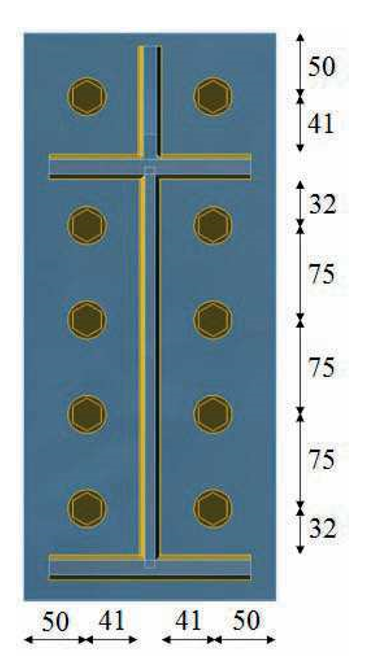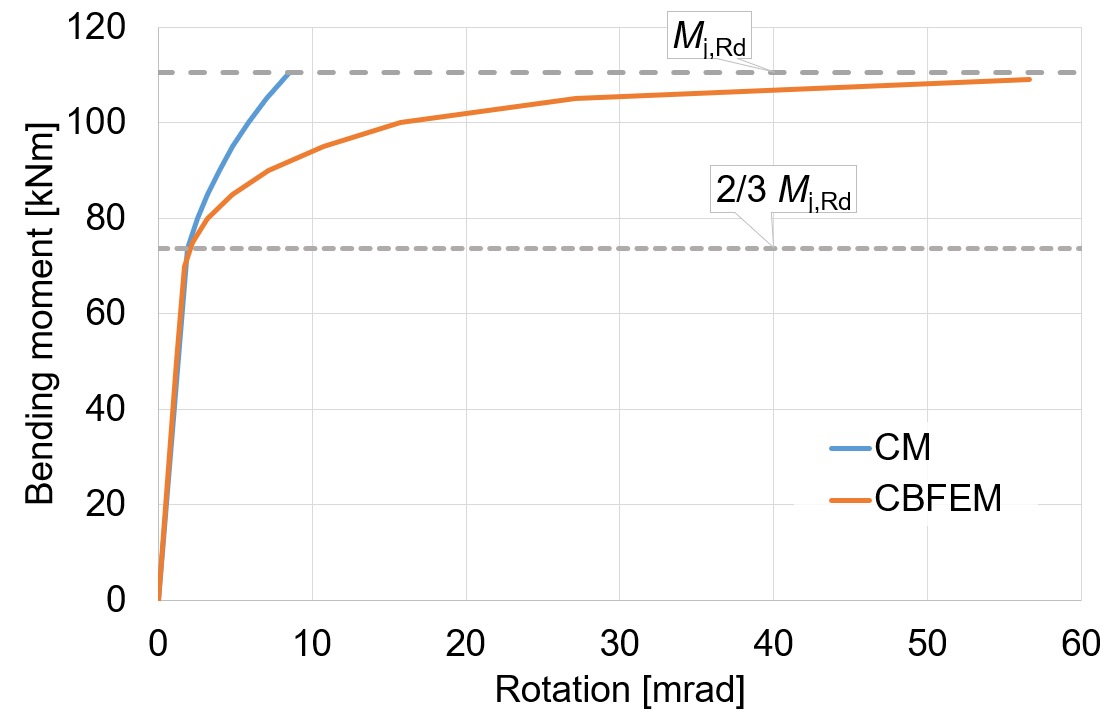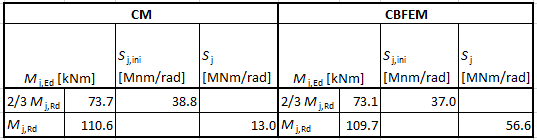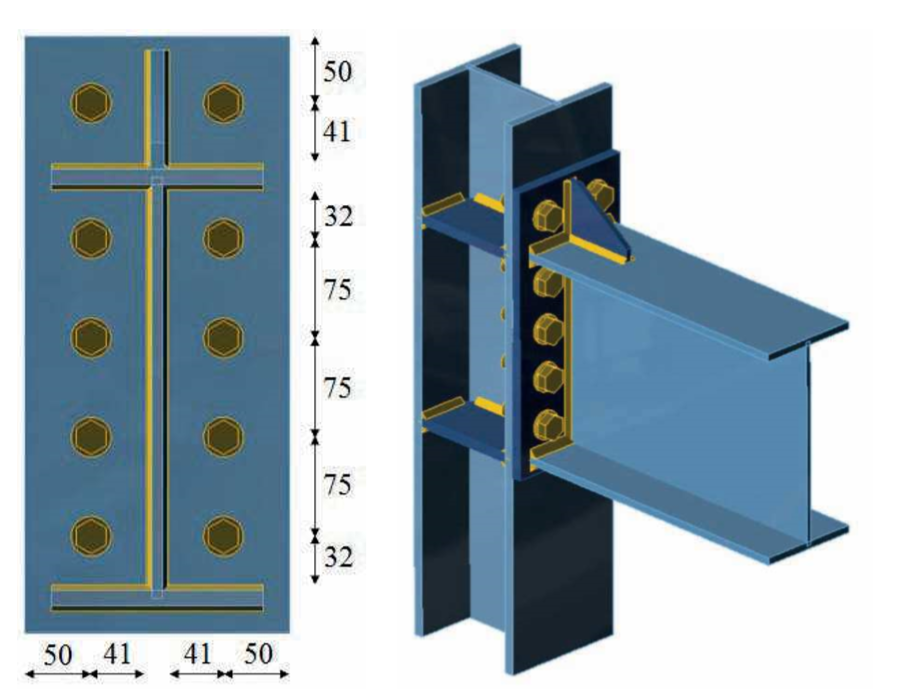# Bending stiffness of bolted joint of open sections

A sample chapter from book Benchmark cases for advanced design of structural steel connections
$$10.2.1 DescriptionThe prediction of rotational stiffness is verified on a bolted eaves moment joint. A bolted joint of open section column HEB and beam IPE is studied and the joint’s behaviour is described on moment-rotation diagram. The results of analytical model by the component-based finite element method (CBFEM) are compared with the component method (CM). The numerical results in form of a benchmark case are available.10.2.2 Analytical modelThe rotational stiffness of a joint should be determined from deformation of its basic components, which represented by the stiffness coefficient ki. The rotational stiffness of the joint Sj is obtained from:$S_j = \frac{E z^2}{\mu \Sigma_i \frac{1}{k_i}}$where ki is the stiffness coefficient for the joint component i; z is the lever arm, see 6.2.7; μ is the stiffness ratio, see 6.3.1. The joint components that are taken into account in this example are column web panel in shear k1 and a single equivalent stiffness coefficient keq for end-plate joint with two or more bolt-rows in tension. The stiffness coefficients are defined in Table 6.11 in EN 1993-1-8:2005. The equivalent stiffness coefficient may be obtained in chapter 6.3.3 in EN 1993-1-8:2005. The initial stiffness Sj,ini is obtained for a moment Mj,Ed ≤ 2/3 Mj,Rd.An open section beam IPE 330 is connected with bolted end-plate to a column HEB 200 in the example. The end-plate thickness is 15 mm, the bolt type is M24 8.8 and the assembly is shown in Fig. 10.2.1. The stiffeners are inside column opposite to beam flanges with thickness of 15 mm. Beam flanges are connected to the end-plate with welds throat thickness of 8 mm. The beam web is connected with weld throat thickness of 5 mm. Plasticity is applied in welds. The material of the beam, column and end-plate is S235. The joint is loaded in bending. The design resistance is limited by the component column web panel in shear. The calculated stiffness coefficients of the basic components, initial stiffness, stiffness by design resistance and rotation of the beam are summarised in Tab. 10.2.1.Tab. 10.2.1 Results of the analytical model (Component method) k1 [-]keq [-]Mj,Rd [kNm]Sj,ini [MNm/rad] 3.256.77110.638.81Fig. 10.2.1 Benchmark case for bolted eaves moment joint (IPE330 to HEB200)10.2.3 Numerical modelDetailed information about the prediction of stiffness in CBFEM may be found in chapter 3.9. For this end-plate joint are results summarized in Tab. 10.2.2. The CBFEM analyses allows to calculate secant rotational stiffness in any stages of loading. The design resistance is reached by 5% plastic strain in a component column web panel in shear.Tab. 10.2.2 Results of CBFEM Mj,Rd [kNm]$$\varphi_j$$ [mrad]Sj [MNm/rad] 501.1942.2 601.4242.2 701.741.2 803.225 907.1712.6 10015.756.3 10956.621.9 10.2.4 Global behavior and verificationA comparison of the global behavior of a bolted eaves moment joint described by the moment-rotation diagram is prepared. The joint is analyzed and the stiffness of the connected beam is calculated. The main characteristic is the initial stiffness calculated by 2/3 Mj,Rd, where Mj,Rd is the design moment resistance of the joint. Mc,Rd stands for a design moment resistance of the analysed beam. The moment-rotation diagram is shown in Fig. 10.2.2.Fig. 10.2.2 Moment-rotation diagram for a bolted eaves moment joint10.2.5 Verification of stiffnessThe rotational stiffness calculated by CBFEM compared with CM. The comparison shows good agreement in initial stiffness and correspondence of joint’s behavior. The calculated stiffness from CBFEM and CM are summarized in Tab. 10.2.3.Tab. 10.2.3 Rotational stiffness of an eaves moment joint in CBFEM and CM10.2.6 Benchmark caseInputsBeam and column Steel S235 Column HEB200 Beam IPE330 Weld Flange weld throat thickness af = 8 mm Web weld throat thickness aw = 5 mm End-plate Thickness tp = 15 mm Height hp = 450 mm Width bp = 200 mm Bolts M24 8.8 Bolts assembly in Fig. 10.2.3 Column stiffeners Thickness ts = 15 mm Width bs = 95 mm Related to beam flange, position upper and lower Weld throat thickness as = 6 mm End-plate stiffener Thickness tst = 10 mm Height hst = 90 mm Weld throat thickness ast = 5 mm Outputs Design resistance Mj,Rd= 109 kNm Load Mj,Ed = 2/3 Mj,Rd = 73 kNm Rotational deformation $$\varphi_c$$ = 1,96 mrad Secant rotational stiffness Sjs = 37,2 MNm/radFig. 10.2.3 Benchmark case for bolted eaves moment joint (IPE330 to HEB200) Sample files Open in Viewer Download$$